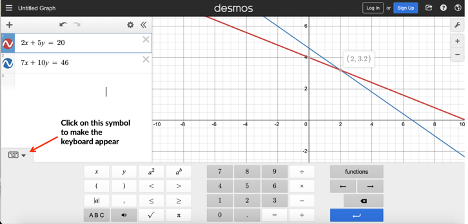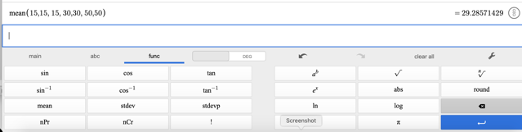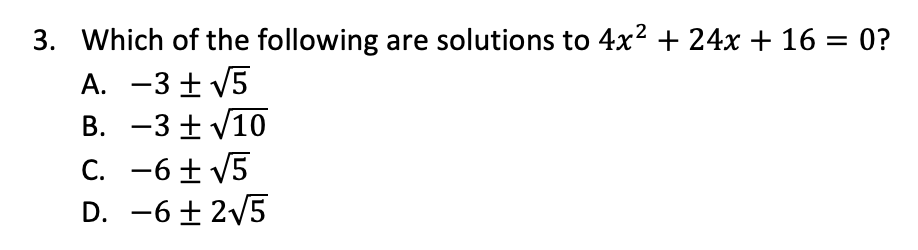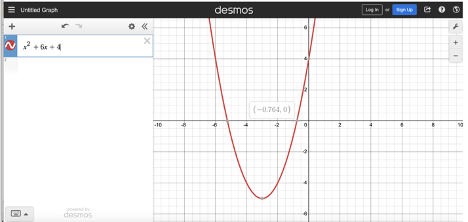# Digital SAT: Learn how to use the new in-app calculatorUpdated June 2023

While you’ll still be able to use your own trusty calculator on the digital SAT, the exam’s new testing app also includes a built-in graphing calculator. The College Board has teamed up with Desmos to make use of the company’s already existing online scientific and graphing calculators. Both calculators are easy to use and include advanced features that come in handy for solving tricky SAT questions.

#### Systems of linear equations

The SAT loves to test your knowledge of systems of equations, so knowing how to approach these questions is vital. Fortunately, you can solve these fairly simply using Desmos’s graphing calculator. For example, you may see a problem such as this one:

2x + 5y = 20
7x + 10y = 46

1. If (xy) is the solution to the system of equations above, what is the value of y?

1. 2
2. 16/5
3. 26/3
4. 6

To solve this problem, first open up Desmos’s graphing calculator. Enter the equations either by typing them in or by using the on-screen keyboard. Once you have typed in the first equation, simply press the “Enter” button, and a space to enter the second equation will appear. The point at which the two equations intersect will be highlighted with a gray dot. Simply click on the dot to make the coordinates of the point appear.Since the solution to a system of equations is the x and y coordinates at which they intersect, the value of y in this case is 3.2. Eliminate choices A and D. Since 26/3 is much larger than 3, you can eliminate choice C. The correct answer is choice B. You can also confirm that this is the correct answer by simply calculating the decimal value of 16/5, which is in fact 3.2.

#### Averages

Questions testing average, or mean, also appear regularly on the SAT, and while you can solve these problems by adding up all of the values in a list of numbers and then dividing by the number of values you have, using Desmos’s average function may be an easy shortcut for problems such as the one below.

 Class Car number Ticket Price Economy 1 − 3 \$15 Business 4 − 6 \$30 Premier 7 − 9 \$50

2. The price of a train ticket depends upon the class of ticket purchased, as shown in the table above. A group plans to purchase 8 train tickets.

They will purchase 3 tickets in car 2

They will purchase 2 tickets in car 5

They will purchase 2 tickets in car 9

What is the average (arithmetic mean) of the cost of the 8 tickets, in dollars? (Round your answer to the nearest one-hundredth.)

To find the answer using Desmos’s scientific calculator, first click on the “func” button, and then press “mean.” Next, enter the list of numbers for which you would like to find the average. Here, each of the tickets in car 2 will cost \$15, each of the tickets in car 5 will cost \$30, and each of the tickets in car 9 will cost \$50, so the list you would enter the list shown below in Desmos.As you can see, the mean is 29.28571429. However, the question indicates that you should round to the nearest one-hundredth, so the correct answer is 29.29.

Quadratics are heavily tested on the SAT, so understanding how to find solutions to quadratic equations using a graphing calculator can be especially helpful. Questions such as the one below are typical of what you might see.To find the solutions, enter the equation into Desmos’ graphing calculator. You should see a graph similar to the one shown here:The x-intercepts will appear as gray dots. Click on the dots to make the coordinates of these points appear. Since the x-intercepts are the points at which the y-value of the equation equals zero, the x-values of these two points are the solutions to the equation. As you can see in the figure above, one of the x-intercepts is -0.764. The other is –0.5236. Simply find the values of each answer choice in decimal form, using the calculator, and look for the choice that gives you –0.764 and –0.5236. You should find that the correct answer is choice A.

In addition to the graphing and average functions, the Desmos calculator will also allow you to find the values of trigonometric functions, calculate probability, and find the absolute value of an expression, among other things. SAT Math may still remain a challenging topic, but knowing how to use the tool at your disposal can make your task much easier!

The recommendations above are general suggestions. If you have specific questions, reach out to our experts here. We’re happy to help in any way we can.